# Marginal distribution with ggplot2 and ggExtra

This post explains how to add marginal distributions to the X and Y axis of a `ggplot2` scatterplot. It can be done using histogram, boxplot or density plot using the `ggExtra` library.

# Basic use of `ggMarginal()`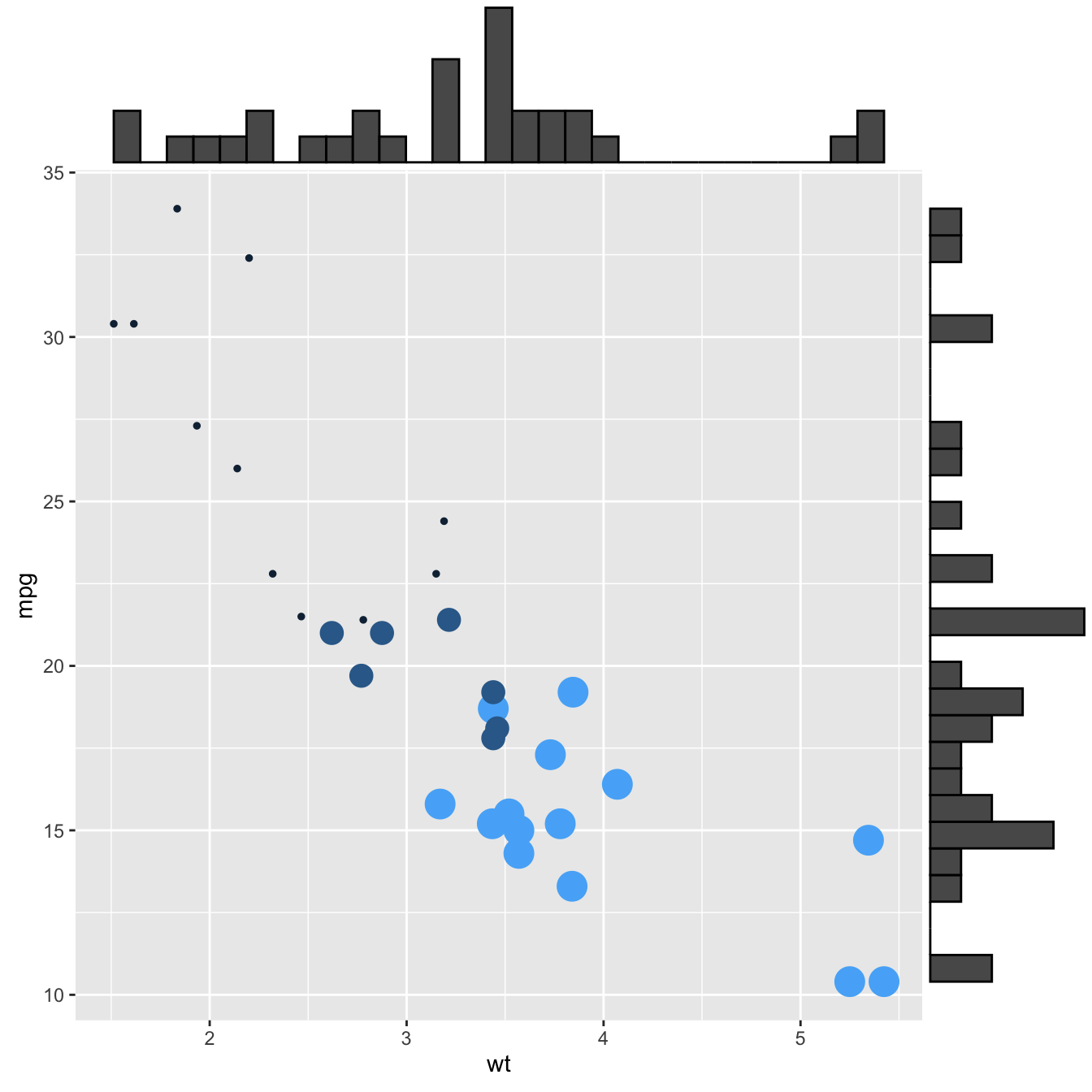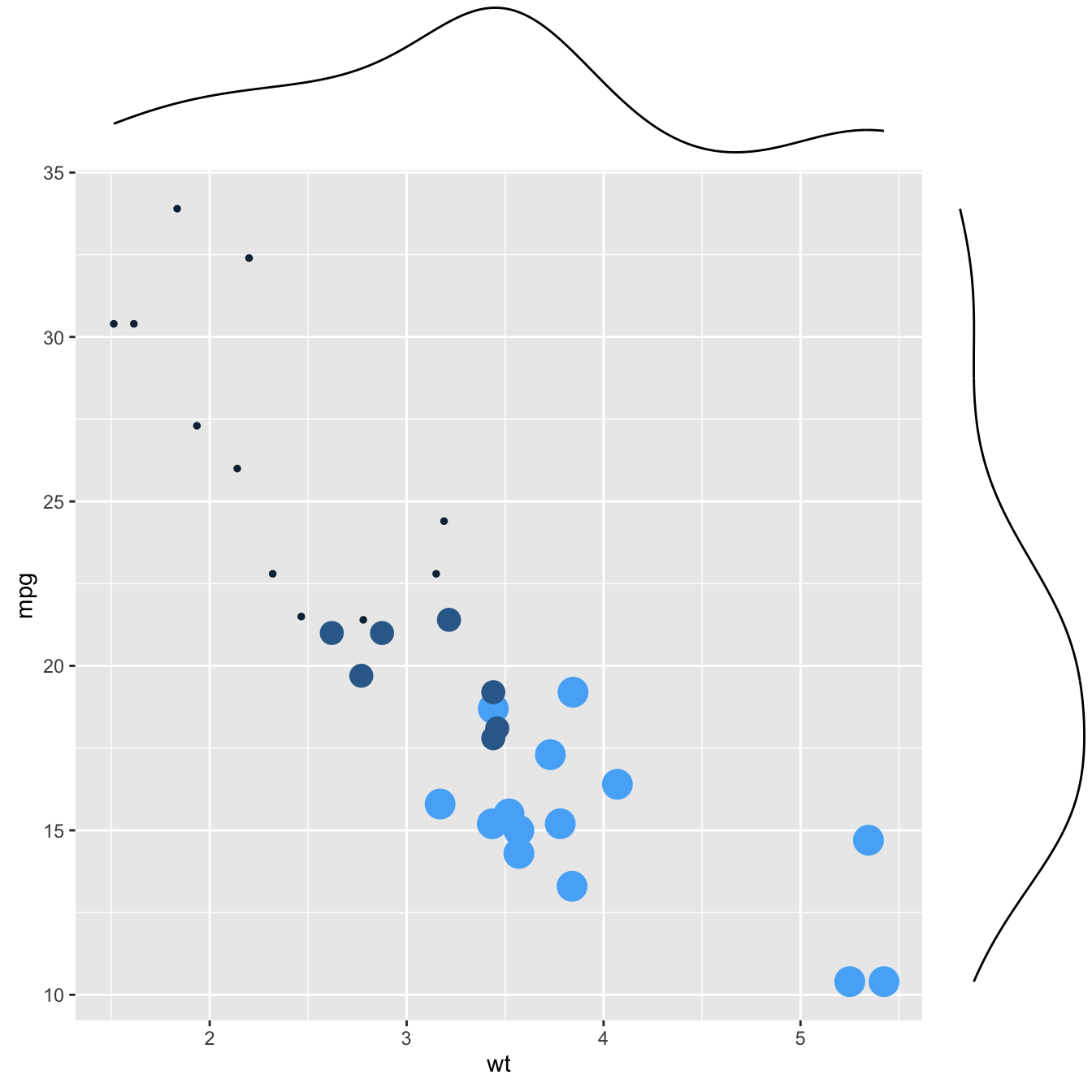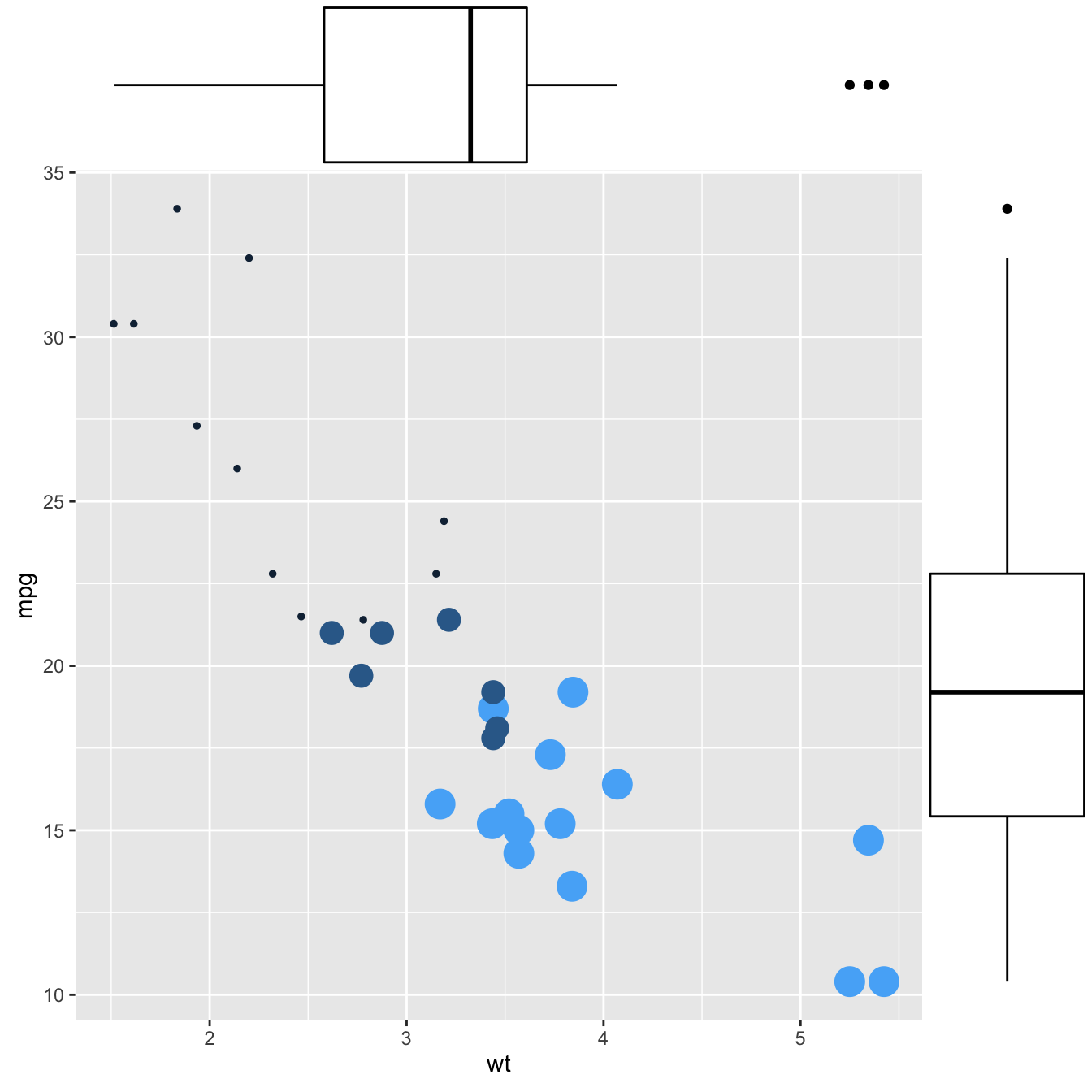Here are 3 examples of marginal distribution added on X and Y axis of a scatterplot. The `ggExtra` library makes it a breeze thanks to the `ggMarginal()` function. Three main types of distribution are available: histogram, density and boxplot.

``````# library
library(ggplot2)
library(ggExtra)

# The mtcars dataset is proposed in R

# classic plot :
p <- ggplot(mtcars, aes(x=wt, y=mpg, color=cyl, size=cyl)) +
geom_point() +
theme(legend.position="none")

# with marginal histogram
p1 <- ggMarginal(p, type="histogram")

# marginal density
p2 <- ggMarginal(p, type="density")

# marginal boxplot
p3 <- ggMarginal(p, type="boxplot")``````

# More customization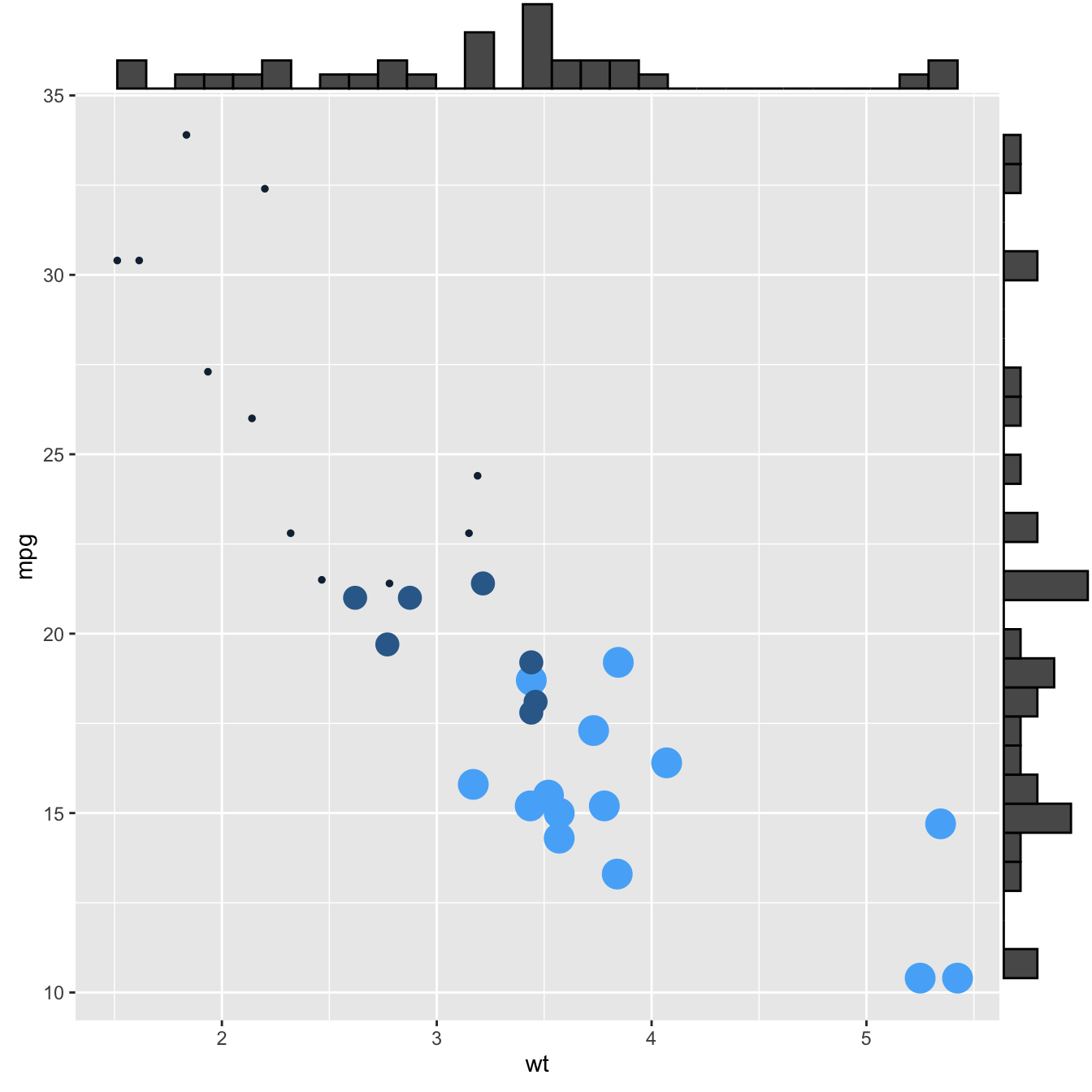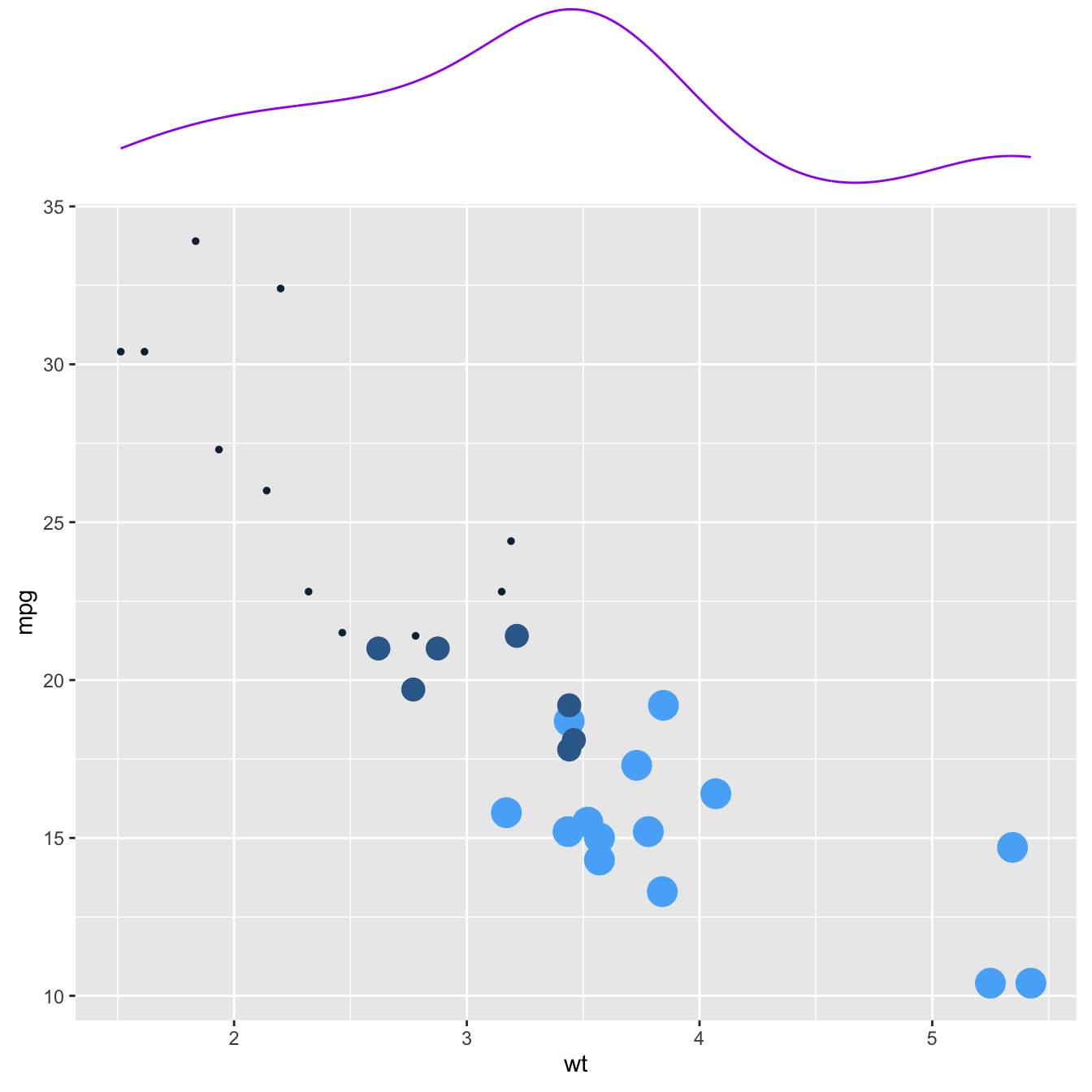Three additional examples to show possible customization:

• change marginal plot size with `size`
• custom marginal plot appearance with all usual parameters
• show only one marginal plot with `margins = 'x'` or `margins = 'y'`
``````# library
library(ggplot2)
library(ggExtra)

# The mtcars dataset is proposed in R

# classic plot :
p <- ggplot(mtcars, aes(x=wt, y=mpg, color=cyl, size=cyl)) +
geom_point() +
theme(legend.position="none")

# Set relative size of marginal plots (main plot 10x bigger than marginals)
p1 <- ggMarginal(p, type="histogram", size=10)

# Custom marginal plots:
p2 <- ggMarginal(p, type="histogram", fill = "slateblue", xparams = list(  bins=10))

# Show only marginal plot for x axis
p3 <- ggMarginal(p, margins = 'x', color="purple", size=4)``````

Related chart types

## Contact

This document is a work by Yan Holtz. Any feedback is highly encouraged. You can fill an issue on Github, drop me a message on Twitter, or send an email pasting yan.holtz.data with gmail.com.